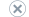# Microsoft Naive Bayes Algorithm Technical Reference

Applies to:SQL Server 2019 and earlier Analysis ServicesAzure Analysis ServicesPower BI Premium

Important

Data mining was deprecated in SQL Server 2017 Analysis Services and now discontinued in SQL Server 2022 Analysis Services. Documentation is not updated for deprecated and discontinued features. To learn more, see Analysis Services backward compatibility.

The Microsoft Naive Bayes algorithm is a classification algorithm provided by Microsoft SQL Server SQL Server Analysis Services for use in predictive modeling. The algorithm calculates the conditional probability between input and predictable columns, and assumes that the columns are independent. This assumption of independence leads to the name Naive Bayes.

## Implementation of the Microsoft Naive Bayes Algorithm

This algorithm is less computationally intense than other Microsoft algorithms, and therefore is useful for quickly generating mining models to discover relationships between input columns and predictable columns. The algorithm considers each pair of input attribute values and output attribute values.

A description of the mathematical properties of Bayes Theorem is beyond the scope of this documentation; for more information, see the paper by Microsoft Research titled Learning Bayesian Networks: The Combination of Knowledge and Statistical Data.

For a description of how probabilities in all models are adjusted to account for potential missing values, see Missing Values (Analysis Services - Data Mining).

### Feature Selection

The Microsoft Naive Bayes algorithm performs automatic feature selection to limit the number of values that are considered when building the model. For more information, see Feature Selection (Data Mining).

Naive Bayes Shannon's Entropy

Bayesian with K2 Prior

Bayesian Dirichlet with uniform prior (default)
Naive Bayes only accepts discrete or discretized attributes; therefore, it cannot use the interestingness score.

The algorithm is designed to minimize processing time and efficiently select the attributes that have the greatest importance; however, you can control the data that is used by the algorithm by setting parameters as follows:

• To limit the values that are used as inputs, decrease the value of MAXIMUM_INPUT_ATTRIBUTES.

• To limit the number of attributes analyzed by the model, decrease the value of MAXIMUM_OUTPUT_ATTRIBUTES.

• To limit the number of values that can be considered for any one attribute, decrease the value of MINIMUM_STATES.

## Customizing the Naive Bayes Algorithm

The Microsoft Naive Bayes algorithm supports several parameters that affect the behavior, performance, and accuracy of the resulting mining model. You can also set modeling flags on the model columns to control how data is processed, or set flags on the mining structure to specify how missing values or nulls should be handled.

### Setting Algorithm Parameters

The Microsoft Naive Bayes algorithm supports several parameters that affect the performance and accuracy of the resulting mining model. The following table describes each parameter.

MAXIMUM_INPUT_ATTRIBUTES
Specifies the maximum number of input attributes that the algorithm can handle before it invokes feature selection. Setting this value to 0 disables feature selection for input attributes.

The default is 255.

MAXIMUM_OUTPUT_ATTRIBUTES
Specifies the maximum number of output attributes that the algorithm can handle before it invokes feature selection. Setting this value to 0 disables feature selection for output attributes.

The default is 255.

MINIMUM_DEPENDENCY_PROBABILITY
Specifies the minimum dependency probability between input and output attributes. This value is used to limit the size of the content that is generated by the algorithm. This property can be set from 0 to 1. Larger values reduce the number of attributes in the content of the model.

The default is 0.5.

MAXIMUM_STATES
Specifies the maximum number of attribute states that the algorithm supports. If the number of states that an attribute has is greater than the maximum number of states, the algorithm uses the attribute's most popular states and treats the remaining states as missing.

The default is 100.

### Modeling Flags

The Microsoft Decision Trees algorithm supports the following modeling flags. When you create the mining structure or mining model, you define modeling flags to specify how values in each column are handled during analysis. For more information, see Modeling Flags (Data Mining).

Modeling Flag Description
MODEL_EXISTENCE_ONLY Means that the column will be treated as having two possible states: Missing and Existing. A null is a missing value.

Applies to mining model column.
NOT NULL Indicates that the column cannot contain a null. An error will result if Analysis Services encounters a null during model training.

Applies to mining structure column.

## Requirements

A Naive Bayes tree model must contain a key column, at least one predictable attribute, and at least one input attribute. No attribute can be continuous; if your data contains continuous numeric data, it will be ignored or discretized.

### Input and Predictable Columns

The Microsoft Naive Bayes algorithm supports the specific input columns and predictable columns that are listed in the following table. For more information about what the content types mean when used in a mining model, see Content Types (Data Mining).

Column Content types
Input attribute Cyclical, Discrete, Discretized, Key, Table, and Ordered
Predictable attribute Cyclical, Discrete, Discretized, Table, and Ordered

Note

Cyclical and Ordered content types are supported, but the algorithm treats them as discrete values and does not perform special processing.